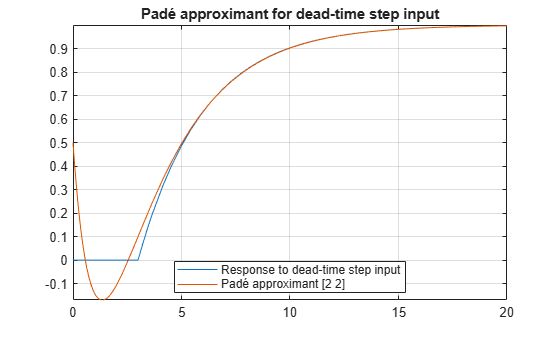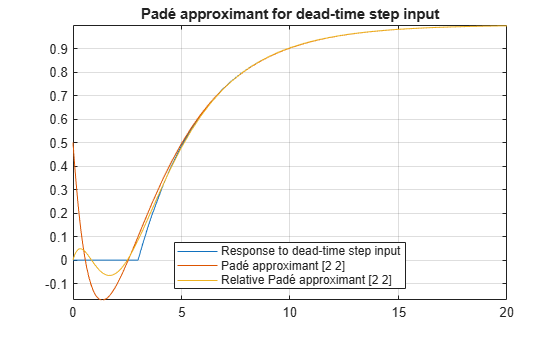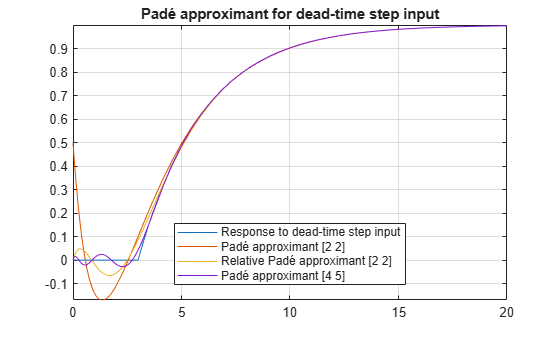This example shows how to use a Padé approximant in control system theory to model time delays in the response of a first-order system. Time delays arise in systems such as chemical and transport processes where there is a delay between the input and the system response. When these inputs are modeled, they are called dead-time inputs.

This example uses Symbolic Math Toolbox™ to solve for the transfer function of a first-order system and find the system response to dead-time step input using Padé approximant. This example performs calculations symbolically to obtain analytic results.

Introduction

The Padé approximant of order [m, n] approximates the function f(x) around $x={x}_{0}$ as

$\frac{{a}_{0}+{a}_{1}\phantom{\rule{0.16666666666666666em}{0ex}}\left(x-{x}_{0}\right)+\cdots +{a}_{m}\phantom{\rule{0.16666666666666666em}{0ex}}\left(x-{x}_{0}{\right)}^{m}}{1+{b}_{1}\phantom{\rule{0.16666666666666666em}{0ex}}\left(x-{x}_{0}\right)+\cdots +{b}_{n}\phantom{\rule{0.16666666666666666em}{0ex}}\left(x-{x}_{0}{\right)}^{n}}.$

The Padé approximant is a rational function formed by a ratio of two power series. Because it is a rational function, it is more accurate than the Taylor series in approximating functions with poles. The Padé approximant is represented by the Symbolic Math Toolbox™ function pade.

When a pole or zero exists at the expansion point $x={x}_{0}$, the accuracy of the Padé approximant decreases. To increase accuracy, use an alternative form of the Padé approximant which is

$\frac{\left(x-{x}_{0}{\right)}^{p}\left({a}_{0}+{a}_{1}\phantom{\rule{0.16666666666666666em}{0ex}}\left(x-{x}_{0}\right)+\cdots +{a}_{m}\phantom{\rule{0.16666666666666666em}{0ex}}\left(x-{x}_{0}{\right)}^{m}\right)}{1+{b}_{1}\phantom{\rule{0.16666666666666666em}{0ex}}\left(x-{x}_{0}\right)+\cdots +{b}_{n}\phantom{\rule{0.16666666666666666em}{0ex}}\left(x-{x}_{0}{\right)}^{n}}.$

The pade function returns the alternative form of the Padé approximant when you set the OrderMode input argument to Relative.

Find Transfer Function of First-Order System

The behavior of a first-order system is described by this differential equation

$\tau \frac{dy\left(t\right)}{dt}+y\left(t\right)=ax\left(t\right).$

Enter the differential equation in MATLAB®.

syms tau a x(t) y(t) xS(s) yS(s) H(s) tmp
F = tau*diff(y)+y == a*x;

Find the Laplace transform of F using laplace.

F = laplace(F,t,s)
F = $\mathrm{laplace}\left(y\left(t\right),t,s\right)-\tau \left(y\left(0\right)-s \mathrm{laplace}\left(y\left(t\right),t,s\right)\right)=a \mathrm{laplace}\left(x\left(t\right),t,s\right)$

Assume the response of the system at t = 0 is 0. Use subs to substitute for y(0) = 0.

F = subs(F,y(0),0)
F = $\mathrm{laplace}\left(y\left(t\right),t,s\right)+s \tau \mathrm{laplace}\left(y\left(t\right),t,s\right)=a \mathrm{laplace}\left(x\left(t\right),t,s\right)$

To collect common terms, use simplify.

F = simplify(F)
F = $\left(s \tau +1\right) \mathrm{laplace}\left(y\left(t\right),t,s\right)=a \mathrm{laplace}\left(x\left(t\right),t,s\right)$

For readability, replace the Laplace transforms of x(t) and y(t) with xS(s) and yS(s).

F = subs(F,[laplace(x(t),t,s) laplace(y(t),t,s)],[xS(s) yS(s)])
F = $\mathrm{yS}\left(s\right) \left(s \tau +1\right)=a \mathrm{xS}\left(s\right)$

The Laplace transform of the transfer function is yS(s)/xS(s). Divide both sides of the equation by xS(s) and use subs to replace yS(s)/xS(s) with H(s).

F = F/xS(s);
F = subs(F,yS(s)/xS(s),H(s))
F = $H\left(s\right) \left(s \tau +1\right)=a$

Solve the equation for H(s). Substitute for H(s) with a dummy variable, solve for the dummy variable using solve, and assign the solution to Hsol(s).

F = subs(F,H(s),tmp);
Hsol(s) = solve(F,tmp)
Hsol(s) =

$\frac{a}{s \tau +1}$

Find Response of System to Time-Delayed Step Input

The input to the first-order system is a time-delayed step input. To represent a step input, use heaviside. Delay the input by three time units. Find the Laplace transform using laplace.

step = heaviside(t - 3);
step = laplace(step)
step =

$\frac{{\mathrm{e}}^{-3 s}}{s}$

Find the response of the system, which is the product of the transfer function and the input.

y = Hsol(s)*step
y =

$\frac{a {\mathrm{e}}^{-3 s}}{s \left(s \tau +1\right)}$

To allow plotting of the response, set parameters a and tau to specific values. For a and tau, choose values 1 and 3, respectively.

y = subs(y,[a tau],[1 3]);
y = ilaplace(y,s);

Find Response of System Using Padé Approximants

Find the Padé approximant of order [2 2] of the step input using the Order input argument to pade.

$\frac{3 {s}^{2}-4 s+2}{2 s \left(s+1\right)}$

Find the response to the input by multiplying the transfer function and the Padé approximant of the input.

$\frac{a \left(3 {s}^{2}-4 s+2\right)}{2 s \left(s \tau +1\right) \left(s+1\right)}$

Find the inverse Laplace transform of yPade22 using ilaplace.

$a+\frac{9 a {\mathrm{e}}^{-s}}{2 \tau -2}-\frac{a {\mathrm{e}}^{-\frac{s}{\tau }} \left(2 {\tau }^{2}+4 \tau +3\right)}{\tau \left(2 \tau -2\right)}$

To plot the response, set parameters a and tau to their values of 1 and 3, respectively.

$\frac{9 {\mathrm{e}}^{-s}}{4}-\frac{11 {\mathrm{e}}^{-\frac{s}{3}}}{4}+1$

Plot the response of the system y and the response calculated from the Padé approximant yPade22.

fplot(y,[0 20])
hold on
grid on
'Location', 'Best');Increase Accuracy of Padé Approximant using OrderMode

The [2 2] Padé approximant does not represent the response well because a pole exists at the expansion point of 0. To increase the accuracy of pade when there is a pole or zero at the expansion point, set the OrderMode input argument to Relative and repeat the steps. For details, see pade.

$\frac{3 {s}^{2}-6 s+4}{s \left(3 {s}^{2}+6 s+4\right)}$

$\frac{a \left(3 {s}^{2}-6 s+4\right)}{s \left(s \tau +1\right) \left(3 {s}^{2}+6 s+4\right)}$

$\frac{12 {\mathrm{e}}^{-t} \left(\mathrm{cos}\left(\frac{\sqrt{3} t}{3}\right)+\frac{2 \sqrt{3} \mathrm{sin}\left(\frac{\sqrt{3} t}{3}\right)}{3}\right)}{7}-\frac{19 {\mathrm{e}}^{-\frac{t}{3}}}{7}+1$Increase Accuracy of Padé Approximant by Increasing Order

You can increase the accuracy of the Padé approximant by increasing its order. Increase the order to [4 5] and repeat the steps. The [n-1 n] Padé approximant is better at approximating the response at t = 0 than the [n n] Padé approximant.

$\frac{27 {s}^{4}-180 {s}^{3}+540 {s}^{2}-840 s+560}{s \left(27 {s}^{4}+180 {s}^{3}+540 {s}^{2}+840 s+560\right)}$

$\frac{a \left(27 {s}^{4}-180 {s}^{3}+540 {s}^{2}-840 s+560\right)}{s \left(s \tau +1\right) \left(27 {s}^{4}+180 {s}^{3}+540 {s}^{2}+840 s+560\right)}$

$\frac{27 {s}^{4}-180 {s}^{3}+540 {s}^{2}-840 s+560}{s \left(3 s+1\right) \left(27 {s}^{4}+180 {s}^{3}+540 {s}^{2}+840 s+560\right)}$

Find the inverse Laplace transform of yPade45 using ilaplace. Approximate yPade45 numerically using vpa. Plot the response calculated from the Padé approximant yPade45.Conclusions

The following points have been shown: